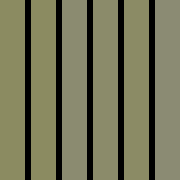## Solution for Net-Force.nl : Level 701 – Hey, where are you from?

Solution for Net-Force.nl : Level 701 – Hey, where are you from?
This is the link to the original challenge: http://www.net-force.nl/challenge/level701/index.php
Quest:
When all the HTTP vars are [OK] you will find the password!
 Forwarded: (politie.nl) [FAILED] OS: (Unix) [FAILED] Via: (net-force.nl) [FAILED]
Tools Required:
Logic Behind this challenge:
Well from initial analysis, it seems that the author of this challenge wanted us to fake our data to conform to what he / she wanted.
So let’s use Modify Headers and fill it with these information as shown in the image below.

After we have started it, and we try to refresh the challenge page. It will look like this.

It seems like we are required to get it right if we use a Mac or Ubuntu. But in this case, i prefer to use User Agent Switcher and changed it to Android user agent.

After changing to that, we smelled success and immediately. The answer appeared right before our eyes.

Cheers
0x4A61636F62

## Solution for Net-Force.nl : Level 601 – Keep walking…

Solution for Net-Force.nl : Level 601 – Keep walking…

This is the link to the original challenge: http://www.net-force.nl/challenge/level601/
Quest:
This is a challenge to test your basic programming skills.

Pseudo code:
Set X = 1
Set Y = 1
Set previous answer = 1

answer = X * Y + previous answer + 3

After that => X + 1 and Y + 1 (‘answer’ becomes ‘previous answer’) and repeat this till you have X = 525.

The final answer is the value of ‘answer’ when X = 525. Fill it in below to check if it’s the correct answer. If it is, you will get the password for the challenge page.

Example:
```5 = 1 * 1 + 1 + 3 12 = 2 * 2 + 5 + 3 24 = 3 * 3 + 12 + 3 ........................ ........................```

Since this is a programming challenge, let’s look at the clues given to us.

Set X = 1
Set Y = 1
Set previous answer = 1

answer = X * Y + previous answer + 3

So we need 3 variables, xy and answer as we can reuse “answer” as “previous answer“. This will translate to something like the following.

x=1
y=1
answer = x * y + answer + 3

Based on the hints given, i’ve finally code an python script that gave me the answer.

import os, sys

def main():
x=1
y=1

for z in range(0,525):
answer = x * y + answer + 3
x+=1
y+=1
if x==524:

if __name__ == ‘__main__’:
main()

Running my own script, i got back this:

previous answer = 47823644

x=526, y=526

Entering the answer in the challenge page and we smelled success again.

So the answer for this challenge is “pr0ggen

Cheers
0x4A61636F62

## Solution for Net-Force.nl : Level 804 – Nice colors eh?

Solution for Net-Force.nl : Level 804 – Nice colors eh?

This is the link to the original challenge: http://www.net-force.nl/challenge/level804/
Quest:
Uhm ok, get the password out of this image…it’s a dutch word 🙂Required Tools:
Gimp –  http://www.gimp.org/
AsciiTable – http://www.asciitable.com/

Logic Behind this Challenge:
Ok, from what i can see right now, the image seems to be in different shades of a similar colour.
Using Gimp, i have derived the following HTML notation for the colours:
8B8B61, 8B8B61, 8B8B70, 8B8B6A, 8B8B65, 8B8B73

As we can see, the HTML notation for all the colours differ slightly. So let’s try converting the last portion of each colour from Hex back to Ascii.

The word that we got back is aapjes and that is the password to this particular challenge.

Cheers
0x4A61636F62